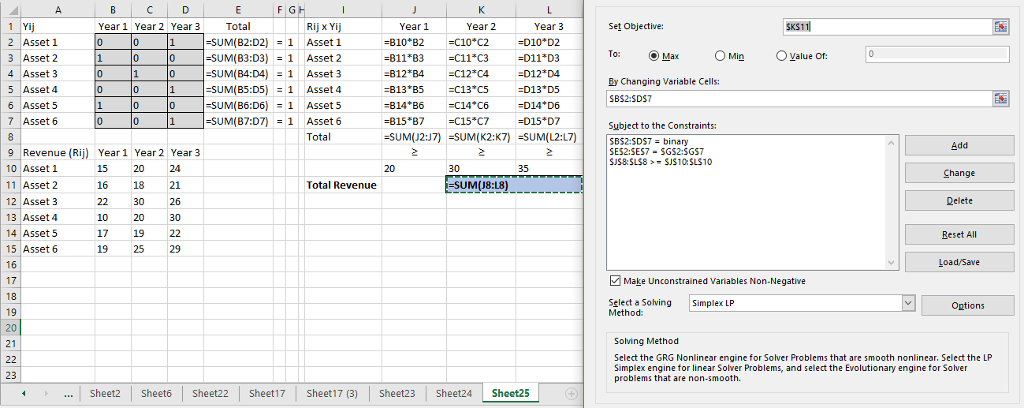# Solved: Boris Milkem owns six assets that he must sell over the next three years. For

Boris Milkem owns six assets that he must sell over the next three years. For example, if he sells asset 3 in year 2, he receives \$30 million. Each asset can be sold at most once, of course. He must sell at least \$20 million of assets in year 1, \$30 million in year 2, and 35 million in year 3. How can he maximize his total revenue from the sales? He expects that the sales will generate the following revenue, in millions of \$

Let Yij be a binary integer so that
Yij = 1 when the i-th Asset is sold in j-th year
=0, otherwise ∀i = {1,2,…,6} and ∀j = {1,2,3}

 Yij Year 1 Year 2 Year 3 Asset 1 Y11 Y12 Y13 Asset 2 Y21 Y22 Y23 Asset 3 Y31 Y32 Y33 Asset 4 Y41 Y42 Y43 Asset 5 Y51 Y52 Y53 Asset 6 Y61 Y62 Y63

Objective Function: max.Z = Total Revenue = ∑i,j Rij x Yij where Rij is the revenue matrix given

Don't use plagiarized sources. Get Your Custom Essay on
Solved: Boris Milkem owns six assets that he must sell over the next three years. For
GET AN ESSAY WRITTEN FOR YOU FROM AS LOW AS \$13/PAGE

Subject to,

1. All assets can be invested once only

• Yi1 + Yi2 + Yi3 = 1 ∀i = {1,2,…,6}

2. Minimum year-wise revenue

• i (Yi1 x Ri1) ≥ 20 ∀i = {1,2,…,6}
• i (Yi2 x Ri2) ≥ 30 ∀i = {1,2,…,6}
• i (Yi3 x Ri3) ≥ 35 ∀i = {1,2,…,6}

3. Binary Integer constraints: Yij ∈ {0,1}

Implementation in excel:Yij Year 1 Year 2 Year 3 Total Rij x Yij Year 1 Year 2 Year 3 Asset 1 0 0 1 1 = 1 Asset 1 0 0 24 Asset 2 1 0 0 1 = 1 Asset 2 16 0 0 Asset 3 0 1 0 1 = 1 Asset 3 0 30 0 Asset 4 0 0 1 1 = 1 Asset 4 0 0 30 Asset 5 1 0 0 1 = 1 Asset 5 17 0 0 Asset 6 0 0 1 1 = 1 Asset 6 0 0 29 Total 33 30 83 Revenue (Rij) Year 1 Year 2 Year 3 ≥ ≥ ≥ Asset 1 15 20 24 20 30 35 Asset 2 16 18 21 Total Revenue 146 Asset 3 22 30 26 Asset 4 10 20 30 Asset 5 17 19 22 Asset 6 19 25 29